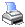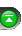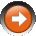﻿ Time Series Forecast (TSF)
 NinjaScript > Language Reference > Indicator Methods > Time Series Forecast (TSF)Description

The Time Series Forecast function displays the statistical trend of a security's price over a specified time period based on linear regression analysis. Instead of a straight linear regression trendline, the Time Series Forecast plots the last point of multiple linear regression trendlines. This is why this indicator may sometimes referred to as the "moving linear regression" indicator or the "regression oscillator."

Syntax

 TSF(int forecast, int period) TSF(IDataSeries input, int forecast, int period)   Returns default value TSF(int forecast, int period)[int barsAgo] TSF(IDataSeries input, int forecast, int period)[int barsAgo]

Return Value

double; Accessing this method via an index value [int barsAgo] returns the indicator value of the referenced bar.

Parameters

 forecast Forecast period input Indicator source data (?) period Number of bars used in the calculation

Examples

 // Prints the current value of a 20 period TSF using default price type double value = TSF(3, 20); Print("The current TSF value is " + value.ToString());

Source Code

You can view this indicator method source code by selecting the menu Tools > Edit NinjaScript > Indicator within the NinjaTrader Control Center window.